Data Structures
Topics:
Basics of Disjoint Data Structures
• Arrays
• Stacks
• Queues
• Hash Tables
• Trees
• Disjoint Data Structures
• Basics of Disjoint Data Structures

# Basics of Disjoint Data Structures

The efficiency of an algorithm sometimes depends on the data structure that is used. An efficient data structure, like the disjoint-set-union, can reduce the execution time of an algorithm.

Assume that you have a set of N elements that are into further subsets and you have to track the connectivity of each element in a specific subset or connectivity of subsets with each other. You can use the union-find algorithm (disjoint set union) to achieve this.

Let’s say there are 5 people A, B, C, D, and E.
A is B's friend, B is C's friend, and D is E's friend, therefore, the following is true:

1. A, B, and C are connected to each other
2. D and E are connected to each other

You can use the union-find data structure to check each friend is connected to the other directly or indirectly. You can also determine the two different disconnected subsets. Here, 2 different subsets are {A, B, C} and {D, E}.

You have to perform two operations:

1. Union(A, B): Connect two elements A and B
2. Find(A, B): Find whether the two elements A and B are connected

Example You have a set of elements S = {0, 1, 2, 3, 4, 5, 6, 7, 8, 9}. Here there are 10 elements (N = 10). Use an array arr to manage the connectivity of the elements. Arr[ ] that is indexed by elements of sets, which are of size N (as N elements in set), can be used to manage the operations of union and find.

Assumption

A and B objects are connected only if arr[ A ] = arr[ B ].

Implementation

To implement the operations of union and find, do the following:

1. Find(A, B): Check whether arr[ A ] = arr[ B ]
2. Union(A, B): Connect A to B and merge the components that comprise A and B by replacing elements that have a value of arr[ A ] with the value of arr[ B ].

Initially there are 10 subsets and each subset has one element in it.When each subset contains only one element, the array arr is as follows: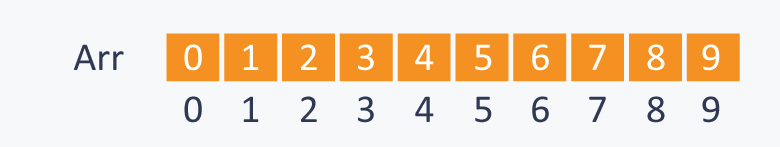Let’s perform some operations.

1. Union(2, 1)The arr is as follows: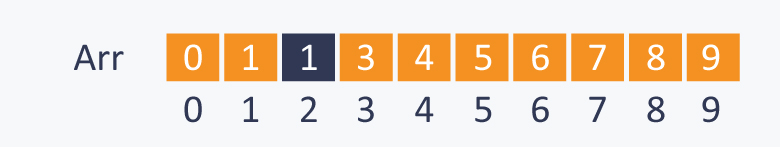1. Union(4, 3)

2. Union(8, 4)

3. Union(9, 3)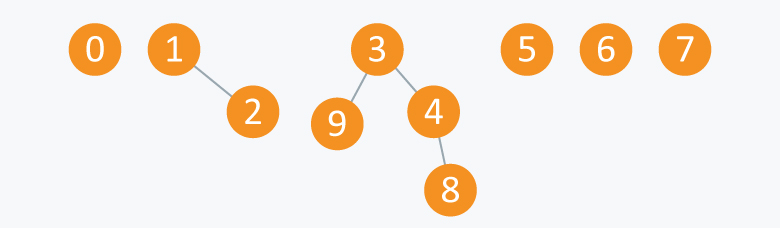The arr is as follows: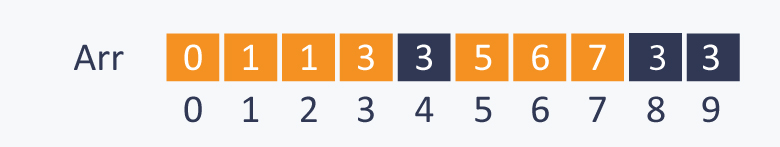1. Union(6, 5)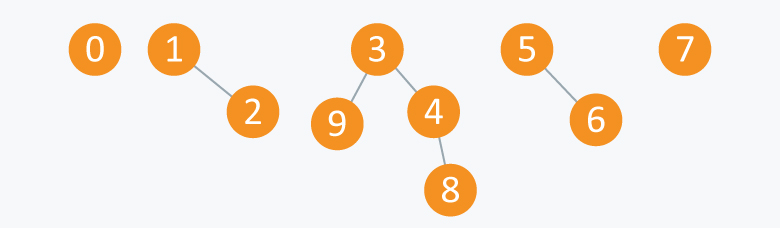The arr is as follows: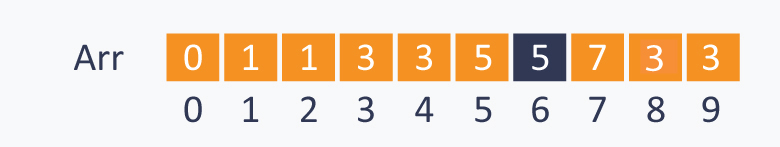After performing some operations of Union (A ,B), there are now 5 subsets as follows:

1. First subset comprises the elements {3, 4, 8, 9}
2. Second subset comprises the elements {1, 2}
3. Third subset comprises the elements {5, 6}
4. Fourth subset comprises the elements {0}
5. Fifth subset comprises the elements {7}

The elements of a subset, which are connected to each other directly or indirectly, can be considered as the nodes of a graph. Therefore, all these subsets are called connected components.

The union-find data structure is useful in graphs for performing various operations like connecting nodes, finding connected components etc.

Let’s perform some find(A, B) operations.

1. Find(0, 7): 0 and 7 are disconnected, and therefore, you will get a false result
2. Find(8, 9): Although 8 and 9 are not connected directly, there is a path that connects both the elements, and therefore, you will get a true result

When these operations are viewed in terms of components, then:

1. Union(A, B): Replace components that comprise the two objects A and B with their union
2. Find(A, B): Check whether the two objects A and B are in the same component

If you perform the operation union(5, 2) on the components mentioned above, then it will be as follows: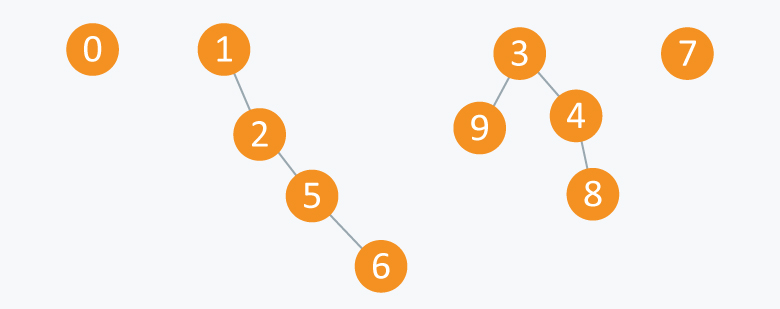The arr is as follows: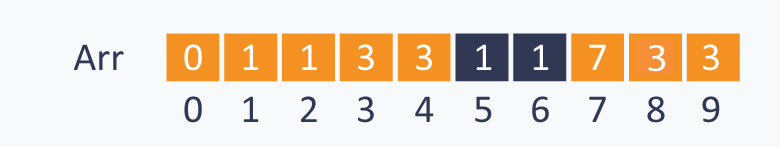Implementation

Approach A

Initially there are N subsets containing one element in each subset. Therefore, to initialize the array use the initialize () function.

    void initialize( int Arr[ ], int N)
{
for(int i = 0;i<N;i++)
Arr[ i ] = i ;
}
//returns true if A and B are connected, else returns false
bool find( int Arr[ ], int A, int B)
{
if(Arr[ A ] == Arr[ B ])
return true;
else
return false;
}
//change all entries from arr[ A ] to arr[ B ].
void union(int Arr[ ], int N, int A, int B)
{
int TEMP = Arr[ A ];
for(int i = 0; i < N;i++)
{
if(Arr[ i ] == TEMP)
Arr[ i ] = Arr[ B ];
}
}


Time complexity (of this approach)

As the loop in the union function iterates through all the N elements for connecting two elements, performing this operation on N objects will take O(N2) time, which is quite inefficient.

Approach B

Let’s try another approach.

Idea

Arr[ A ] is a parent of A.

Consider the root element of each subset, which is only a special element in that subset having itself as the parent. Assume that R is the root element, then arr[ R ] = R.

For more clarity, consider the subset S = {0, 1, 2, 3, 4, 5}

Initially each element is the root of itself in all the subsets because arr[ i ] = i, where i is the element in the set. Therefore root(i) = i.Performing union(1, 0) will connect 1 to 0 and will set root (0) as the parent of root (1). As root(1) = 1 and root(0) = 0, the value of arr[ 1 ] will change from 1 to 0. Therefore, 0 will be the root of the subset that contains the elements {0, 1}.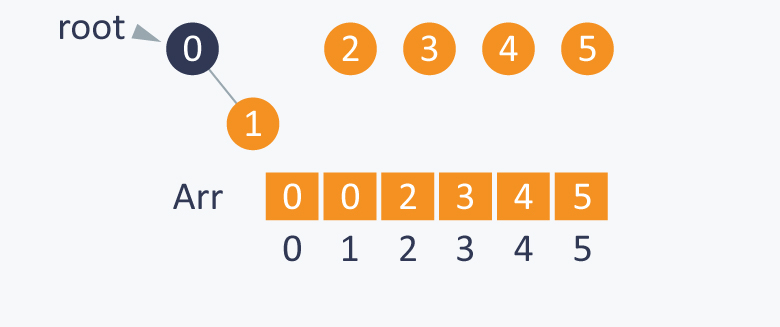Performing union (0, 2), will indirectly connect 0 to 2 by setting root(2) as the parent of root(0). As root(0) is 0 and root(2) is 2, it will change value of arr[ 0 ] from 0 to 2. Therefore, 2 will be the root of the subset that contains the elements {2, 0, 1}.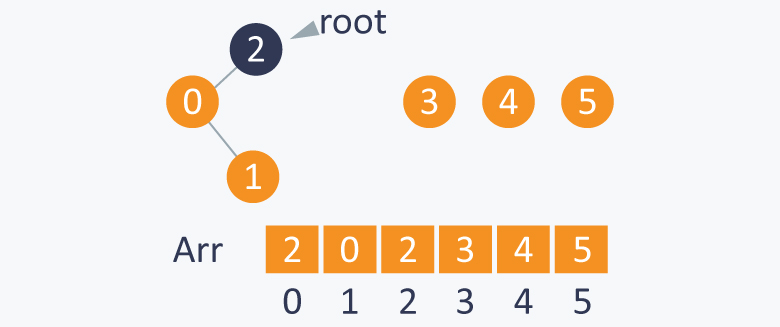Performing union (3, 4) will indirectly connect 3 to 4 by setting root(4) as the parent of root(3). As root(3) is 3 and root(4) is 4, it will change the value of arr[ 3 ] from 3 to 4. Therefore, 4 will be the root of the subset that contains the elements {3, 4}.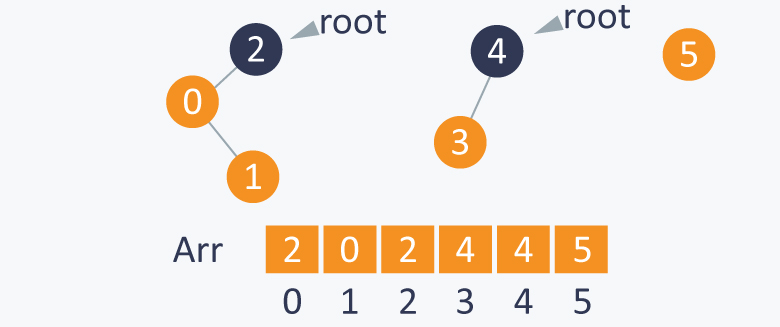Performing union (1, 4) will indirectly connect 1 to 4 by setting root(4) as the parent of root(1). As root(4) is 4 and root(1) is 2, it will change value of arr[ 2 ] from 2 to 4. Therefore, 4 will be the root of the set containing elements {0, 1, 2, 3, 4}.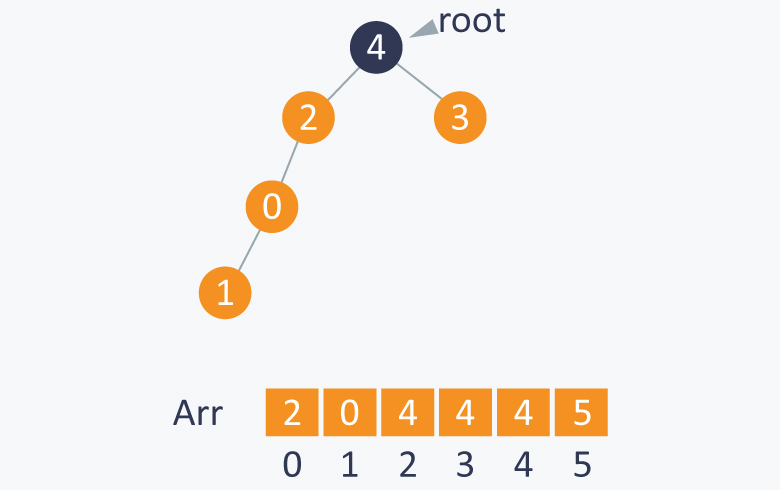After each step, you will see the change in the array arr also.

After performing the required union(A, B) operations, you can perform the find(A, B) operation easily to check whether A and B are connected. This can be done by calculating the roots of both A and B. If the roots of A and B are the same, then it means that both A and B are in the same subset and are connected.

Calculating the root of an element

Arr[ i ] is the parent of i (where i is the element of the set). The root of i is Arr[ Arr[ Arr[ …...Arr[ i ]...... ] ] ] until arr[ i ] is not equal to i. You can run a loop until you get an element that is a parent of itself.

Note This can only be done when there is no cycle in the elements of the subset, else the loop will run infinitely.

1. Find(1, 4): 1 and 4 have the same root i.e. 4. Therefore, it means that they are connected and this operation will give the result True.

2. Find(3, 5): 3 and 5 do not have same root because root(3) is 4 and root(5) is 5. This means that they are not connected and this operation will give the result False.

Implementation

Initially each element is a parent of itself, which can be done by using the initialize function as discussed above.

  //finding root of an element
int root(int Arr[ ],int i)
{
while(Arr[ i ] != i)           //chase parent of current element until it reaches root
{
i = Arr[ i ];
}
return i;
}

/*modified union function where we connect the elements by changing the root of one of the elements*/

int union(int Arr[ ] ,int A ,int B)
{
int root_A = root(Arr, A);
int root_B = root(Arr, B);
Arr[ root_A ] = root_B ;       //setting parent of root(A) as root(B)
}
bool find(int A,int B)
{
if( root(A)==root(B) )       //if A and B have the same root, it means that they are connected.
return true;
else
return false;
}

In the worst case, this idea will also take linear time in connecting 2 elements and determining (finding) whether two elements are connected. Another disadvantage is that while connecting two elements, which subset has more elements is not checked. This may sometimes create a big problem because you will have to perform approximately linear time operations.

To avoid this, track the size of each subset and while connecting two elements connect the root of each subset that has a smaller number of elements to the root of each subset that has a larger number of elements.

Example

If you want to connect 1 and 5, then connect the root of subset A (the subset that contains 1) to the root of subset B ( the subset that contains 5) because subset A contains less number of elements than subset B.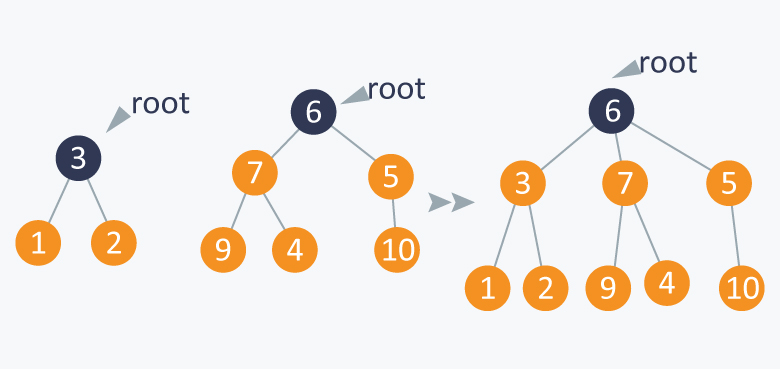It will balance the tree formed by performing the operations discussed above. This is known as weighted-union operation .

Implementation

Initially the size of each subset will be one because each subset will have only one element. You can initialize it in the initialize function discussed above. The size[ ] array function will keep a track of the size of each subset.

  //modified initialize function:
void initialize( int Arr[ ], int N)
{
for(int i = 0;i<N;i++)
{
Arr[ i ] = i ;
size[ i ] = 1;
}
}


The root() and find() functions will be same as discussed above .

The union function will be modified because the two subsets will be connected based on the number of elements in each subset.

//modified union function

void weighted-union(int Arr[ ],int size[ ],int A,int B)
{
int root_A = root(A);
int root_B = root(B);
if(size[root_A] < size[root_B ])
{
Arr[ root_A ] = Arr[root_B];
size[root_B] += size[root_A];
}
else
{
Arr[ root_B ] = Arr[root_A];
size[root_A] += size[root_B];
}

}


Example

You have a set S = {0, 1, 2, 3, 4, 5} Initially all the subsets have a single element and each element is a root of itself. Initially size[ ] array will be as follows:Perform union(0, 1). Here, you can connect any root of any element with the root of another element because both the element’s subsets are of the same size. After the roots are connected, the respective sizes will be updated. If you connect 1 to 0 and make 0 the root, then the size of 0 will change from 1 to 2.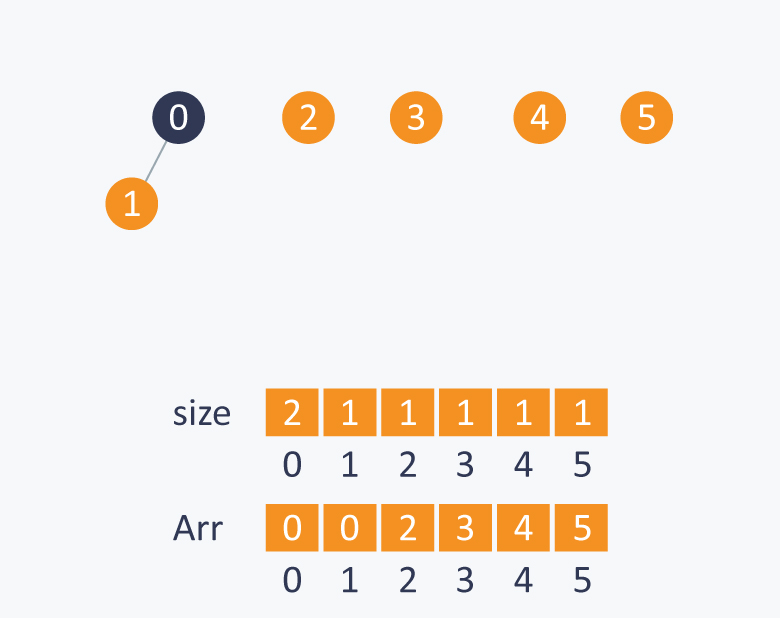While performing union(1, 2), connect root(2) with root(1) because the subset of 2 has fewer elements than the subset of 1.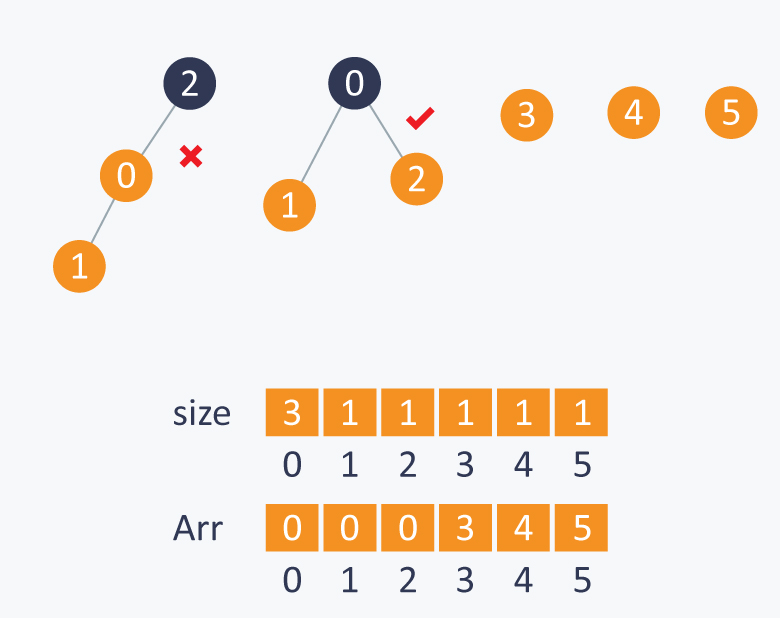Similarly, in union(3, 2), connect root(3) to root(2) because the subset of 3 has fewer elements than the subset of 2.Maintaining a balance tree, will reduce complexity of the union-find function from N to log2N.

Idea for improving this approach further

Union with path compression

While computing the root of A, set each i to point to its grandparent (thereby halving the length of the path), where i is the node that comes in the path while computing the root of A.

//modified root function

int root (int Arr[ ] ,int i)
{
while(Arr[ i ] != i)
{
Arr[ i ] = Arr[ Arr[ i ] ] ;
i = Arr[ i ];
}
return i;
}


When you use the weighted-union operation with path compression it takes log * N for each union find operation, where N is the number of elements in the set.

log *N is the iterative function that computes the number of times you have to take the log of N before the value of N reaches 1.

Contributed by: Prateek Garg